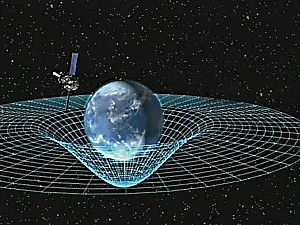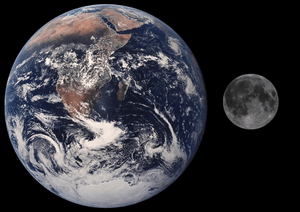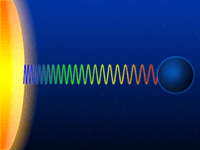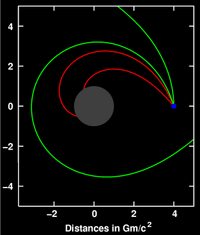# Gravity facts for kids

Kids Encyclopedia Facts
(Redirected from Gravitation)Artist concept of Gravity Probe B orbiting the Earth to measure space-time, a four-dimensional description of the universe including height, width, length, and time.

Gravity, or gravitation, is one of the fundamental forces of the universe. In this article, we discuss it in three parts:

1. Everyday sense: the force which causes objects to fall onto the ground.
2. Newton's laws: how gravity keeps the Solar System together.
3. Einstein's theory of general relativity: the role of gravity in the universe.

Some physicists think gravity is caused by gravitons, but they are still unsure.

## Everyday gravity

### Weight vs massThe Moon is much smaller than the earth so the pull of gravity on the Moon is about 1/6th that of Earth.

In everyday talk, we say things fall because the Earth's gravity pulls on them. We talk as if our weight was a "given". Actually, weight changes when the pull of gravity changes. The Moon is much smaller and the pull of gravity on the Moon is about 1/6th that of Earth. So any object on the Moon weighs 1/6th of its weight on Earth. What does not change is the amount of matter in an object. That is called its mass. On Earth, mass and weight are the same for practical purposes. The trip to the Moon shows they are not really the same.

From this we learn two things.

1. The weight of an object is variable; its mass is constant.
2. The pull of gravity varies according to the mass of an object. The Earth pulls more strongly than the Moon. A person also exerts a gravitational pull, but it is so tiny it can be ignored for all practical purposes.

The Earth has mass. Every particle of matter has mass. So the Earth pulls on every object and person and they pull on the Earth. Gravity pulls on the mass and gives it weight.

### Acceleration due to gravity

The acceleration gained by an object because of gravitational force is called its acceleration due to gravity.

The acceleration due to gravity at the surface of Earth is represented as g.

#### Why heavier objects do not fall faster

Isaac Newton deduced that the resultant force equals mass times acceleration. The bigger the mass of the falling object, the greater the force of gravitational attraction pulling it towards Earth. However, the bigger mass cancels out the greater force of attraction, so the acceleration is the same.

A common experiment to see this is to drop a feather and a bowling ball from the same height. To see the effect of gravity you need to remove all the air from the room so you don't get any air resistance (a vacuum). Both the feather and the bowling ball will reach the bottom at the same time, despite the feather being lighter and the bowling ball being heavier.

### Gravity vs gravitation

These words mean almost the same thing in everyday use. Sometimes scientists use "gravity" for the force that pulls objects towards each other, and "gravitation" for the theory about the attraction.

## History of gravitational theory

### Galileo

In the late 16th century, Galileo did a famous experiment about gravity where he dropped balls from the Tower of Pisa. He later rolled balls down inclines. With these experiments, Galileo showed that gravitation accelerates all objects at the same rate regardless of weight.

### Kepler

The man who really worked it all out was Johannes Kepler, who, after "900 pages of calculations and many false starts and dead-end ideas", finally got these three laws:

1. Kepler's elliptical orbit law: The planets orbit the sun in elliptical orbits with the sun at one focus.
2. Kepler's equal-area law: The line connecting a planet to the sun sweeps out equal areas in equal amounts of time.
3. Kepler's law of periods: The time required for a planet to orbit the sun, called its orbital period, is proportional to the long axis of the ellipse raised to the 3/2 power. The constant of proportionality is the same for all the planets.

### Newton

In 1687, English mathematician Isaac Newton wrote the Principia. In this book, he wrote about the inverse-square law of gravitation. Newton said that the closer two objects are to each other, the more gravity will affect them. His theory about gravitation was used to predict the existence of the planet Neptune based on changes in the orbit of Uranus.

Newton's theory was later used to predict the existence of another planet closer to the Sun than Mercury. When this was done, it was learned that his theory was not entirely correct. These mistakes in his theory were corrected by Albert Einstein's theory of General Relativity. Newton's theory is still commonly used for many things because it is much more simple to work with than the theory of General Relativity and is usually accurate enough for many uses.

### Dynamic equilibrium

Why does the Earth not fall into the Sun? The answer is simple but very important. It is because the Earth moving round the Sun is in a dynamic equilibrium. The speed of the Earth's movement creates a centrifugal force which balances the gravitational force between the Sun and the Earth. Why does the Earth continue spinning? Because there is no force to stop it.

Newton's first law: "If a body is at rest it remains at rest or if it is in motion it moves at the same speed until it is acted on by an external force".

There is a kind of analogy between centrifugal force and gravitational force, which led to the "equivalence principle" of general relativity.

#### Weightlessness

In free fall a thing's motion balances out the pull of gravity on it. This includes being in orbit.

## General relativity

The special theory of relativity describes systems where gravity is not an issue; by contrast, gravity is the central issue of the general theory of relativity.

In general relativity there is no gravitational force deflecting objects from their natural, straight paths. Instead, gravity is seen as changes in the properties of space and time. In turn, this changes the straightest-possible paths that objects will naturally follow. The curvature is, in turn, caused by the energy–momentum of matter. Spacetime tells matter how to move; matter tells spacetime how to curve.

For weak gravitational fields and slow speeds relative to the speed of light, the theory's predictions converge on those of Newton's law of universal gravitation. Newton's equations are used to plan journeys in our Solar System.

General relativity has a number of physical consequences.

### Gravitational time dilation and frequency shiftSchematic representation of the gravitational redshift of a light wave escaping from the surface of a massive body

Gravity influences the passage of time. Light sent down into a gravity well is blueshifted, whereas light sent in the opposite direction (i.e., climbing out of the gravity well) is redshifted; collectively, these two effects are known as the gravitational frequency shift.

More generally, processes close to a massive body run more slowly when compared with processes taking place farther away; this effect is known as gravitational time dilation.

### Light deflection and gravitational time delayDeflection of light (sent out from the location shown in blue) near a compact body (shown in gray)

General relativity predicts that the path of light is bent in a gravitational field; light passing a massive body is deflected towards that body. This effect has been confirmed by observing the light of stars or distant quasars being deflected as it passes the Sun.

Closely related to light deflection is the gravitational time delay (or Shapiro delay), the phenomenon that light signals take longer to move through a gravitational field than they would in the absence of that field. There have been numerous successful tests of this prediction.

A parameter called γ encodes the influence of gravity on the geometry of space.

### Gravitational waves

Gravitational waves are ripples in the curvature of spacetime. They move as a wave, travelling outward from the source. Einstein predicted them in 1915 on the basis of his theory of general relativity. In theory, gravitational waves transport energy as gravitational radiation. Sources of detectable gravitational waves might include binary star systems composed of white dwarfs, neutron stars, or black holes. In general relativity, gravitational waves cannot travel faster than the speed of light.

Although gravitational radiation has not been directly detected, there is indirect evidence for its existence. The 1993 Nobel Prize in Physics was awarded for measurements of the Hulse-Taylor binary star system. These measurements suggest gravitational waves are more than mathematical peculiarities. Various gravitational wave detectors exist. However, they have not yet detected the phenomena.

## Images for kidsGravity Facts for Kids. Kiddle Encyclopedia.